# Important Questions For You!

Chapter 1: Electric Charges and Field

1. In which orientation, a dipole placed in a uniform electric field is in (i) stable, (ii) unstable equilibrium?                               

2. Define the term 'dielectric constant' of a medium in terms of capacitance of a capacitor.                                                     

3. Define electric flux. Write its S.I. Units. A spherical rubber balloon carries a charge that is uniformity distributed over its surface. As the balloon is blown up and increases in size, how does the total electric flux coming out of the surface change? Give reason. 

4. An electric dipole of length 4 cm when placed with its axis making an angle of 60° with a uniform electric field, experiences a torque ofCalculate the potential energy of the dipole if it has charge ± 8 nC.                                                                 

5. State Gauss’s theorem . Derive an expression for the electric field due to a charged plane sheet. Find the potential difference between the plates of a parallel plate capacitor having surface density of charge 5 x 10-8 cm-2 with the separation between plates being 4mm                                                                                                                                                                       

Chapter 2: Electrostatic Potential and Capacitance

1. What is the geometrical shape of equipotential surfaces due to a single isolated charge?                                                     

2. For any charge configuration, equipotential surface through a point is normal to the electric field. Justify.                             

3. A parallel plate capacitor of capacitance C is charged to a potential V. It is then connected to another uncharged capacitor having the same capacitance. Find out the ratio of the energy stored in the combined system to that stored initially in the single capacitor.                                                                                                                                                                                         
4. A capacitor of unknown capacitance is connected across a battery of V volts. The charge stored in it is 300 μC. When potential across the capacitor is reduced by 100 V, the charge stored in it becomes 100 μC. Calculate the potential V and the unknown capacitance. What will be the charge stored in the capacitor if the voltage applied had increased by 100 V?                             

5. A parallel plate capacitor is charged by a battery. After sometime the battery is disconnected and a dielectric slabs its thickness equal to the plate separation is inserted between the plates. How will (i) the capacitance of the capacitor. (ii) Potential difference between the plates and (iii) the energy stored in the capacitor be affected?                                                                          

6. Draw a labelled diagram of Van de Graaff generator. State its working principle to show how by introducing a small charged sphere into a larger sphere, a large amount of charge can be transferred to the outer sphere. State the use of this machine and also point out its limitations.                                                                                                                                            

7. Derive an expression for capacitance of parallel plate capacitor with dielectric slab of thickness t(t<d) between the plates separated by distance d. If the dielectric slab is introduced with the battery connected, then how do the following quantities change(i) charge (ii) potential (iii) Capacitance (iv) Energy.

8.

1. Deduce the expression for the potential energy of a system of two charges q1 and q2 locatedand, respectively, in an external electric field.
2. Three point charges, +Q, +2Q and –3Q are placed at the vertices of an equilateral triangle ABC of side ‘l’. If these charges are displaced to the mid-point A1, B1 and C1, respectively, find the amount of the work done in shifting the charges to the new locations.Chapter 3: Current Electricity

1. Define the term ‘mobility’ of charge carriers. Write its S.I. unit.                                                                                     

2. Write the mathematical relation between mobility and drift velocity of charge carriers in a conductor. Name the mobile charge carriers responsible for conduction of electric current in (i) an electrolyte (ii) an ionized gas.                                               

3. How much current will an electric bulb draw from 220 V source if the resistance of the bulb is 1200Ω? If in place of bulb, a heater of resistance 100 Ω is connected to the sources, calculate the current drawn by it.                                                   

4. When 5 V potential difference is applied across a wire of length 0.1 m, the drift speed of electrons is 2.5 ×10-4 m/s. If the electron density in the wire is 8 × 1028 m-3, calculate the resistivity of the material of the wire.                                           

5. State the two Kirchhoff’s rules used in electric networks. How are there rules justified?                                                    

6. State Kirchhoff’s rules of current distribution in an electrical network. Using these rules determine the value of the current I1 in the electric circuit given below.7. A number of identical cells, n, each of emf E, internal resistance r connected in series are charged by a d.c. source of emf E′, using a resistor R. Draw the circuit arrangement. Deduce the expressions for (a) the charging current and (b) the potential difference across the combination of the cells.                                                                                                                   

8.

1. Calculate the resistance of 1 km long copper wire of radius 1 mm. Resistivity of the copper is 1.72 x 10-8 Ω m.
2. Draw a schematic diagram of a circuit consisting of a battery of 4 cells of 2V each connected to a key, an ammeter and two resistors of 2 Ω and 3 Ω respectively in series and a voltmeter to measure potential difference across 3 Ω.                 

Chapter 4: Moving Charges and Magnetism

1. Using the concept of force between two infinitely long parallel current carrying conductors, define one ampere of current.   

2. Write the relation for the forceacting on a charge carrier q moving with a velocity through a magnetic fieldin vector notation. Using this relation, deduce the conditions under which this force will be (i) maximum (ii) minimum.                        

3. A circular coil of closely wound N turns and radius r carries a current I. Write the expressions for the following :                

1. the magnetic field at its centre
2. the magnetic moment of this coil

4. State Biot – Savart law. Deduce the expression for the magnetic field at a point on the axis of a current carrying circular loop of radius ‘R’ distant ‘x’ from the centre. Hence, write the magnetic field at the centre of a loop.                                                

5.

1. Write the expression for the magnetic force acting on a charged particle moving with velocity v in the presence of magnetic field B.
2. A neutron, an electron and an alpha particle moving with equal velocities, enter a uniform magnetic field going into the plane of the paper as shown. Trace their paths in the field and justify your answer.6.

1. Draw a labelled diagram of a moving coil galvanometer. Describe briefly its principle and working.
1. Why is it necessary to introduce a cylindrical soft iron core inside the coil of a galvanometer?
2. Increasing the current sensitivity of a galvanometer may not necessarily increase its voltage sensitivity. Explain, giving reason.                                                                                                                                                          

7. State Biot-Savart law, giving the mathematical expression for it.
Use this law to derive the expression for the magnetic field due to a circular coil carrying current at a point along its axis.
How does a circular loop carrying current behave as a magnet?                                                                                     

Chapter 5: Magnetism and Matter

1. The horizontal component of the Earth’s magnetic field at a place is B and the angle of dip is 60°. What is the value of the vertical component of Earth’s magnetic field?                                                                                                               

2. Where on the surface of Earth is the vertical com-ponent of Earth’s magnetic field zero?

3. Write two characteristics of a material used for making permanent magnets.                                                              

4. The motion of copper plate is damped when it is allowed to oscillate between the two poles of a magnet. What is the cause of this damping?                                                                                                                                                            

5. Define magnetic susceptibility of a material. Name two elements, one having positive susceptibility and the other having negative susceptibility. What does negative susceptibility signify?                                                                                   

6. (a) Draw the magnetic field lines due to a circular loop areacarrying current I. Show that it acts as a bar magnet of magnetic moment. Derive the expression for the magnetic field due to a solenoid of length ‘2l’, radius ‘a’ having ’n’ number of turns per unit length and carrying a steady current ‘I’ at a point on the axial line, distance ‘r’ from the centre of the solenoid. How does this expression compare with the axial magnetic field due to a bar magnet of magnetic moment ‘m’?                                  

Chapter 6: Electromagnetic Induction
1. A conducting loop is held below a current carrying wire PQ as shown. Predict the direction of the induced current in the loop when the current in the wire is constantly increasing.2. A metallic rod of 'L' length is rotated with an angular frequency of 'w' with one end hinged at the center and the other end at the circumference of a circular metallic ring of radius L, about an axis passing through the centre and perpendicular to the plane of the ring. A constant and uniform magnetic field B parallel to the axis is present everywhere. Deduce the expression for the emf between the centre and the metallic ring.                                                                                                                        

3. State Lenz’s Law. A metallic rod held horizontally along east-west direction, is allowed to fall under gravity. Will there be an e.m.f. induced at its ends? Justify your answer.                                                                                                                

4. A metallic rod of length l is rotated at a constant angular speed ω, normal to a uniform magnetic field B. Derive an expression for the current induced in the rod, if the resistance of the rod is R.                                                                                         

5.

1. A rod of length l is moved horizontally with a uniform velocity 'v' in a direction perpendicular to its length through a region in which a uniform magnetic field is acting vertically downward. Derive the expression for the emf induced across the ends of the rod.
2. How does one understand this motional emf by invoking the Lorentz force acting on the free charge carriers of the conductor? Explain.                                                                                                                                           

6.                                                                                                                                                                                    

1. What are eddy currents? Write their two applications.
2. Figure shows a rectangular conducting loop PQSR in which an RS of length ‘l’ is movable. The loop is kept in a uniform magnetic field ‘B’ directed downward perpendicular to the plain of the loop. The arm RS is moved with a uniform speed ‘v’.Deduce an expression for

1. The emf induced across the arm ‘RS’,
2. The external force required to move the arm, and
3. The power dissipated as heat.

7.

1. Define self-inductance of a coil. Obtain an expression for the energy stored in a solenoid of self-inductance ‘L’ when the current though it grows from zero to ‘I’.
2. A square loop MNOP of side 20 cm is placed horizontally in a uniform magnetic field acting vertically downwards as shown in the figure. The loop is pulled with a constant velocity of 20 cm s−1 till it goes out of the field.1. Depict the direction of the induced current in the loop as it goes out of the field. For how long would the current in the loop persist?
2. Plot a graph showing the variation of magnetic flux and induced emf as a function of time.                                      

Chapter 7: Alternating Current
1. The instantaneous current and voltage of an AC circuit are given by I =(10 sin 300t) A and V= (200 sin 300t) V. What is the power dissipation in the circuit?                                                                                                                                    

2. Why is the use of a.c. voltage preferred over d.c. voltage? Give two reasons.                                                               

3. Derive an expression of the impedance of an ac circuit consisting of an inductor and a resistor.                                      

4. A voltage V = V0 sin ωt is applied to a series LCR circuit. Derive the expression for the average power dissipated over a cycle. Under what condition is (i) no power dissipated even though the current flows through the circuit, (ii) maximum power dissipated in the circuit?                                                                                                                                                                  

5. The figure shows a series LCR circuit with L = 5.0 H, C = 80 𝝁F, R = 40 ohm connected to a variable frequency 240 V source. Calculate1. The angular frequency of the source which drives the circuit at resonance.
2. The current at the resonating frequency.
3. The rms potential drop across the capacitor at resonance.

6. The given circuit diagram shows a series LCR circuit connected to a variable frequency 230 V source:1. Determine the source frequency, which drives the circuit in resonance.
2. Obtain the impedance of the circuit and the amplitude of current at the resonating frequency.
3. Determine the rms potential drops across the three elements of the circuit.
4. How do you explain the observation that the algebraic sum of the voltage of the three elements obtained in (c) is greater than the supplied voltage?

Chapter 8: Electromagnetic Waves

1. To which part of the electromagnetic spectrum does a wave of frequency 5 x 1011 Hz belong?                                    

2. Name the EM waves used to studying crystal structure of solids. What is its frequency range?                                     

3. Explain briefly how electromagnetic waves are produced by an oscillating charge? How is the frequency of the electromagnetic waves produced related to that of the oscillating charge?                                                                                             

4. Draw a sketch of a plane electromagnetic wave propagating along the Z-direction. Depict clearly the directions of electric and magnetic fields varying sinusoidally with Z                                                                                                                  

5. What is space wave propagation? State the factors which limit its range of propagation. Derive an expression for the maximum line of sight distance between two antennas for space wave propagation.                                                                       

Chapter 9: Ray Optics and Optical Instruments

1. A glass lens of refractive index 1.5 is placed in a trough of liquid. What must be the refractive index of the liquid in order to make the lens disappear?                                                                                                                                          

2. A convex lens of refractive index 1.5 has a focal length of 18 cm in air. Calculate the change in its focal length when it is immersed in water of refractive index.                                                                                                                   

3. The radii of curvature of the faces of a double convex lens are 10 cm and 15 cm. If focal length of the lens is 12 cm, find the refractive index of the material of the lens.                                                                                                                    

4. A ray of light, incident on an equilateral glass prismmoves parallel to the base line of the prism inside it. Find the angle of incidence for this ray.                                                                                                                                       

5. A convex lens of focal length 20 cm is placed coaxially with a convex mirror of radius of curvature 20 cm. The two are kept 15 cm apart. A point object is placed 40 cm in front of the convex lens. Find the position of the image formed by this combination. Draw the ray diagram showing the image formation.                                                                                                        

6. An object is placed 15 cm in front of a convex lens of focal length 10 cm. Find the nature and position of the image formed. Where should a concave mirror of radius of curvature 20 cm be placed so that the final image is formed at the position of the object itself?                                                                                                                                                                           

7.

1. A biconvex lens has a focal length 2/3 times the radius of curvature of either surface. Calculate the refractive index of lens material.
2. A convex lens of focal length 10 cm is placed coaxially 5 cm away from a concave lens of focal length 10 cm. If an object is placed 30 cm in front of the convex lens find the position of the final image formed by the combined system.            

8. Trace the rays of light showing the formation of an image due to a point object placed on the axis of a spherical surface separating the two media of refractive indices n1 and n2. Establish the relation between the distances of the object, the image and the radius of curvature from the central point of the spherical surface.
Hence derive the expression of the lens maker’s formula .                                                                                                  

Chapter 10: Wave Optics

1. What type of wave front will emerge from a

1. point source
2. distant light source?                                                                                                                                           

2. How the angular separation of interference fringes in Young’s double slit experiment would change when the distance between the slits and screen is halved?                                                                                                                                        

3. How will the interference pattern in Young’s double slit experiment get affected, when

1. distance between the slits S1 and S2 reduced and
2. the entire set up is immersed in water? Justify your answer in each case                                                                 

4. In Young’s double slit experiment, monochromatic light of wavelength 600 nm illuminates the pair of slits and produces an interference pattern in which two consecutive bright fringes are separated by 100 mm. Another source of monochromatic light produces the interference pattern in which the two consecutive bright fringes are separated by 89 mm. Find the wavelength of light from the second source.
What is the effect on the interference fringes if the monochromatic source is replaced by a source of white light?                  

5. The intensity at the central maxima (O) in a Young’s double slit experiment id Io. If the distance OP equals one-third of fringe width of the pattern, then show that the intensity at point P would be Io/4.                                                                        

6. What are coherent sources? Why are coherent sources required to produce interference of light? Give an example of interference of light in everyday life.
In Young’s double slit experiment, the two slits are 0.03 cm apart and the screen is placed at a distance of 1.5 m away from the slits. The distance between the central bright fringe and fourth bright fringe is 1 cm. Calculate the wavelength of light used.    

7. State Huygen’s principle. Show, with the help of a suitable diagram, how this principle is used to obtain the diffraction pattern by a single slit.
Draw a plot of intensity distribution and explain clearly why the secondary maxima become weaker with increasing order (n) of the secondary maxima.                                                                                                                                                           

Chapter 11: Dual Nature of Matter and Radiation

1. The stopping potential in an experiment on photoelectric effect is 2 V. What is the maximum kinetic energy of the photoelectrons emitted?                                                                                                                                                                           

2. Show graphically, the variation of the de-Broglie wavelength (λ) with the potential (V) through which an electron is acceleration from rest.                                                                                                                                                                         

3. A proton and an α-particle are accelerated through the same potential difference. Which one of the two has (i) greater de-Broglie wavelength, and (ii) less kinetic energy? Justify your answer.                                                                                                

4. Plot a graph showing the variation of stopping potential with the frequency of incident radiation for two different photosensitive materials having work functions w1 and w2 (w1 > w2). On what factors does the (i) slope and (ii) intercept of the lines depend? 

5.                                                                                                                                                                                         

1. Why photoelectric effect cannot be explained on the basis of wave nature of light? Give reasons.
2. Write the basic features of photon picture of electromagnetic radiation on which Einstein’s photoelectric equation is based

6. Write Einstein's photoelectric equation. State clearly how this equation is obtained using the photon picture of electro-magnetic radiation. Write the three salient features observed in photoelectric effect which can be explained using this equation.              

Chapter 12: Atoms

1. What is the ratio of radii of the orbits corresponding to first excited state and ground state in a hydrogen atom?                  

2. Using Bohr's postulates of the atomic model derive the expression for radius of nth electron orbit. Hence obtain the expression for Bohr’s radius.                                                                                                                                                              

3. Use Rydberg formula to determine the wavelength of Hα line. [Given: Rydberg constant R = 1.03 × 107m−1]                      

4.

1. Using Bohr’s second postulate of quantization of orbital angular momentum show that the circumference of the electron in the nth orbital state in hydrogen atom is n times the de-Broglie wavelength associated with it.
2. The electron in hydrogen atom is initially in the third excited state. What is the maximum number of spectral lines which can be emitted when it finally moves to the ground state?                                                                                       

5. The energy levels of a hypothetical atom are shown below. Which of the shown transitions will result in the emission of a photon of wavelength 275 nm?
Which of these transitions correspond to emission of radiation of (i) maximum and (ii) minimum wavelength?Chapter 13: Nucle

1. Two nuclei have mass numbers in the ratio 27: 125. What is the ratio of their nuclear radii?                                              

2. State the reason, why heavywater is generally used as a moderator in a nuclear reactor.                                                 

3. A heavy nucleus X of mass number 240 and binding energy per nucleon 7.6 MeV is split into two fragments Y and Z of mass numbers 110 and 130. The binding energy of nucleons in Y and Z is 8.85 MeV per nucleon. Calculate the energy Q released per fission in MeV.                                                                                                                                                                 

4. Explain giving necessary reactions, how energy is released during
(i)fission (ii) fusion                                                                                                                                                           

5. In a typical nuclear reaction,e.galthough number of nucleons is conserved yet energy is released. How? Show that nuclear density in a given nucleus is independent of mass number A.                                                          

6. Define the O-value of a nuclear process. When can a nuclear process not proceed spontaneously? If both the number of protons and the number of neutrons are conserved in a nuclear reaction in what way is mass converted into energy (or vice-versa) in the nuclear reaction?                                                                                                                                                             

Chapter 14: Semiconductor Electronics

1. The output of an OR gate is connected to both the inputs of a NAND gate. Draw the logic circuit of this combination of gates and write its truth table.                                                                                                                                                      

2. In a transistor, doping level in base is increased slightly. How will it affect (i) collector current and (ii) base current?         

3. What is an intrinsic semiconductor? How can this material be converted into (i) P- type (ii) N- type extrinsic semiconductor? Explain with the help of energy band diagrams.                                                                                                                

4. Draw the circuit diagram of an illuminated photodiode in reverse bias. How is photodiode used to measure light intensity?  

5. What is an intrinsic semiconductor? How can this material be converted into (i) P- type (ii) N- type extrinsic semiconductor? Explain with the help of energy band diagrams.                                                                                                                 

6. Give a circuit diagram of a common emitter amplifier using an n – p transistor. Draw the input and output waveforms of the signal. Write the expression for its voltage gain.                                                                                                                

7. State the principle of working of p-n diode as a rectifier. Explain, with the help of a circuit diagram, the use of p-n diode as a full wave rectifier. Draw a sketch of the input and output waveforms.                                                                                        

CASE BASED QUESTIONS

1.Coulomb measured the force between two point charges and found that it varied inversely as the square of the distance between the charges and was directly proportional to the product of the magnitude of the two charges and acted along the line joining the two charges. Thus, if two point charges q1 q2are separated by a distance r in vacuum, the magnitude of the force (F) between them is given by . Consider three charges q1,q2,q3 each equal to q at the vertices of an equilateral triangle of side l. A charge of magnitude Q and polarity same as other charges is placed at the centroid of the triangle.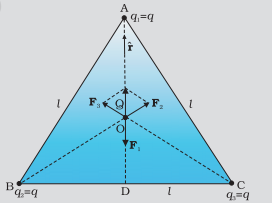a) Force exerted on Q by each charge present on the vertices of the triangle

1. Zero
2. Equal
3. FA >FB >FC
4. FA <FB <FC

b) The magnitude of Force on Q by charge q present on A is

1.2.3.4.c) The magnitude and direction of force experienced by the charge Q due the charge present on the vertex C of the triangle

1. Zero
2.3.4.d) What would be the direction of the Force experienced by the charge q present on vertex A of the triangle due to all other charges.

1. upwards
2. downwards
3. left
4. Right

e) What would be the Resultant force on Q if the polarity of charges present on the vertices in opposite to that of Q

1. Zero
2.3.4.2.In Young’s double slit experiment A monochromatic light is passed through a single narrow slit and falls on S1 and S2.These two slit acts as sources of light waves that interfere constructively and destructively at different points on the screen to produce a pattern of alternating bright and dark fringes. For constructive interferencewhereas for destructive interference. What is the effect on the interference fringes in a Young’s double-slit experiment due to each of the following operations

a) When the screen is moved away from the plane of the slits

1. Separation of fringes increases.
2. Separation of fringes decreases.
3. Separation of fringes remains constant.
4. None of these

b) When the (monochromatic) source is replaced by another (monochromatic) source of shorter wavelength

1. Separation of fringes increases.
2. Separation of fringes decreases.
3. Separation of fringes remains constant.
4. None of these

c) When the separation between the two slits is increased

1. Separation of fringes increases.
2. Separation of fringes decreases.
3. Separation of fringes remains constant.
4. None of these

d) When the source slit is moved closer to the double-slit plane

1. Separation of fringes increases
2. Separation of fringes decreases.
3. Separation of fringes remains constant
4. None of these

e) When the width of the source slit is increased

1. Separation of fringes increases
2. Separation of fringes decreases
3. Separation of fringes remains constant
4. None of these

3. A capacitor is a system of two conductors separated by an insulator. Its capacitance is defined by C = q/V, where q and –q are the charges on the two conductors and V is the potential difference between them. C is determined purely geometrically, by the shapes, sizes and relative positions of the two conductors. The unit of capacitance is farad:,
1 F = 1 C V-1For a parallel plate capacitor (with vacuum between the where A is the area of each plate and d the separation between them)

a) If the medium between the plates of a capacitor is filled with an insulating substance (dielectric), having dielectric constant K, then new capacitance C’ would be

1. C=K2C
2.3.4. C=KC
b) If the medium between the plates of a capacitor is filled with an insulating substance (dielectric), having dielectric constant K, and the battery remains connected, what would be the value of potential difference across the capacitor
1. V
2. V/K
3. KV
4. K2V

c) If the medium between the plates of a capacitor is filled with an insulating substance (dielectric), having dielectric constant K, and the battery remains connected, what would be the value of final charge on the capacitor

1. q/K
2. q/K2
3. Kq
4. q

d) If the medium between the plates of a capacitor is filled with an insulating substance (dielectric), having dielectric constant K, and the battery remains connected, what would be the value of electric field between the plates, (initially the value of electric field is E)

1. E
2. KE
3. K2E
4. E/K

e) If the medium between the plates of a capacitor is filled with an insulating substance (dielectric), having dielectric constant K, and the battery remains connected, what would be the value of energy stored between the plates of the capacitor( initially the value of energy stored between the plates is U)

1. U/K
2. KU
3. K2U
4. U

4. The figure given below depicts a schematic view of the arrangement used for the experimental study of the photoelectric effect .The plate A can be maintained at a desired positive or negative potential with respect to emitter C. When the collector plate A is positive with respect to the emitter plate C, the electrons are attracted to it. The emission of electrons causes flow of electric current in the circuit. The potential difference between the emitter and collector plates is measured by a voltmeter (V) whereas the resulting photo current flowing in the circuit is measured by a microammeter (µA). The photoelectric current can be increased or decreased by varying the potential of collector plate A with respect to the emitter plate C. The intensity and frequency of the incident light can be varied, as can the potential difference V between the emitter C and the collector A.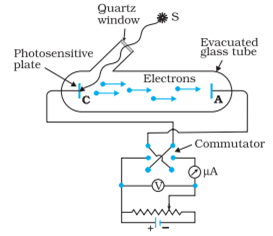Answer the following questions based on the above mention experiment of photoelectric current.
a) On keeping the frequency of the incident radiation and the potential fixed, if the intensity of incident radiation increases, the photoelectric current will

1. Increases
2. Decreases
3. Remains constant
4. None of these

b) For a given frequency of the incident radiation, the stopping potential will

1. Increases with increase in intensity’
2. Decreases with increase in intensity
3. Be independent of intensity
4. None of these

c) If the order of frequency of radiation is V01 > V02>V03, what would be the correct order of stopping potential

1. V01>V02>V03
2. V01<V02<V03
3. V01=V02=V03
4. None of these

d) The emission of photoelectron below the threshold frequency

1. Increases with increase in intensity
2. Decreases with increase in intensity
3. No emission of photoelectron takes place
4. None of these

e) The minimum energy required to remove an electron is called

1. Stopping potential
2. Kinetic energy
3. Work function
4. None of these

5. A series LCR circuit is connected to an a.c. source of variable frequency. A suitable phasor dagram for the amplitude of the current and phase angle has been mentioned below. Answer the following questions based on the concept of LCR circuit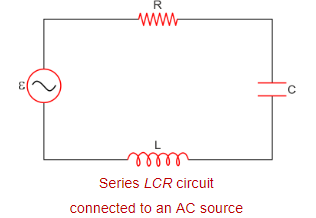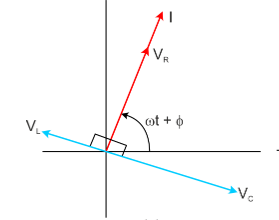1. If Xc> XL, and φ is positive, the circuit is predominantly

1. Inductive
2. Capacitive
3. Neutral
4. None of these

2. If Xc< XL, and φ is negative, the circuit is predominantly

1. Inductive
2. Capacitive
3. Neutral
4. None of these

3. At resonant frequency

1.2.3.4. None of these

4. At resonant frequency the impedance of the LCR circuit is

1. Maximum
2. Minimum
3.4. None of these

5. The ratiois also called the quality factor, Q of the circuit .The larger the value of Q

1. The sharper the resonance
2. The less sharp the resonance
3. Resonance is independent of the value of Q
4. None of these

6. Drift velocity is defined as the average velocity with which free electrons gets drifted in a direction opposite to that of electric field .Let’s say m is the mass of the electron and e be the charge of electron then acceleration a=eE/m. As per the first equation of motion V=u+at. The average initial velocity u=0 and final velocity v=Vd, time taken t=τ(relaxation time) then Vd=a τ. On substituting the value of a we get Vd=eE τ/m. Because of the drift, there will be net transport of charges across any area perpendicular to E. Consider a planar area A, located inside the conductor such that the normal to the area is parallel to E . Then because of the drift, in an infinitesimal amount of time ∆t, all electrons to the left of the area at distances upto | Vd |∆t would have crossed the area. If n is the number of free electrons per unit volume in the metal, then there are n ∆t | Vd |A such electrons. Since each electron carries a charge –e, the total charge transported across this area A to the right in time ∆t is –ne A| Vd |∆t. E is directed towards the left and hence the total charge transported along E across the area is negative of this. The amount of charge crossing the area A in time ∆t is by definition I ∆t, where I is the magnitude of the current. Hence,

I∆t=neAVd∆t

I=nAVd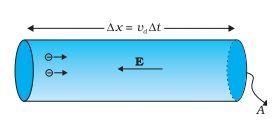I=neAVd
Now consider the following situation,Potential difference V is applied across the ends of copper wire of length l and diameter D.
1. When V is doubled the drift velocity of electron gets

1. Half
2. Doubled
4. Remain unchnged

2. When length(l) is doubled the drift velocity of electron gets

1. Half
2. Doubled
4. Remain Unchanged

3. When the diameter D is doubled the drift velocity of electron gets

1. Half
2. Doubled
4. Remain Unchanged

4. Mobility’ of charge carriers in a conductor

1.2.3.4. None of these

5. With increases in temperature the drift velocity of electrons in a metallic conductor will

1. Decreases
2. Increases
3. Remains constant
4. None of these

7. Consider a ray of light that travels from a denser medium to rarer medium. As the angle of incidence increases in the denser medium the angle of refraction in the rarer medium increases. The angle of incidence for which the angle of refraction becomes 900 is called critical angle.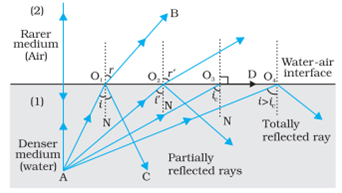When the angle of incidence of a ray travelling from a denser medium to a rarer medium is greater than the critical angle , no refraction occurs. The incident ray is totally reflected back into the same medium. Here, the laws of reflection hold good. Some light is also reflected before critical angle is achieved but not totally.

1. Find the maximum angle that can be made in glass medium (µ=1.5) if a light ray is refractd from glass to vacuum

1.2.3.4. None of these

2. Following are the application of Total Internal Reflection , EXCEPT:

1. Brilliance of diamond
2. Phenomenon Mirage
3. Transmission of signals using optical fiber
4. Dispersion produced by thin prism

3. Which of the following is the necessary condition for the Phenomenon of Total Internal Reflection:

1. Light must incident on the interface from denser medium.
2. Angle of incidence must be greater than critical angle.
3. Angle of incidence is such that angle of refraction is 900
4. Both a and b

4. Considering the figure shown in the passage, when light travels from denser medium to rarer medium, the ray become parallel to the surface after refraction when

1. i=ic
2. i=900
3. i=r
4. i>ic

5. Considering the figure shown in the passage, when light travels from denser medium to rarer medium, the maximum deviation of ray is achieved at

1. i=ic
2. i=900
3. i=r
4. None of these

8. When a small amount of a suitable impurity is added to the pure semiconductor, the conductivity of the semiconductor is increased manifold. Such materials are known as extrinsic semiconductors The deliberate addition of a desirable impurity is called doping and the impurity atoms are called dopants. Such a material is also called a doped semiconductor.There are two types of dopants used in doping the tetravalent Si or Ge:
(i) Pentavalent (valency 5); like Arsenic (As), Antimony (Sb), Phosphorous (P), etc
(ii) Trivalent (valency 3); like Indium (In), Boron (B), Aluminium (Al), etc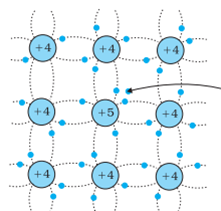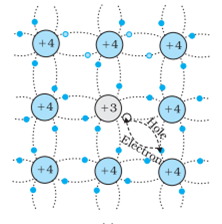n-type                                                                       p-type
When phosphorus atom with five outer electron is substituted for a silicon atom an extra electron is made available for conduction. This addition increases conductivity and the semiconductor is called n-type semiconductor. On the other hand when aluminum atom having three valence electron is substituted for a silicon atom, a hole is created in the valence band and this type of semiconductor is called p-type semiconductor.

1. Let np and ne be the number of wholes and conduction electron in an extrinsic semiconductor then

1. np > ne
2. np < ne
3. np = ne
4. np ≠ ne
Solution d)

2. When an impurity is doped into an intrinsic semiconductor, the conductivity of semiconductor

1. Increases
2. Decreases
3. Remains the same
4. Becomes Zero.

3. The electrical conductivity of intrinsic semiconductor can be increased by

1. Doping acceptor impurities
2. Doping donor impurities
3. Increasing the temperature
4. All of these

4. The impurity atoms with which pure silicon may be doped to make it a p-type semiconductor are those of

1. Boron
2. Antimony
3. Aluminum
4. Both a and c

5. A semiconductor is doped with a donor impurity

1. The hole concentration decreases
2. The The hole concentration increases
3. electron concentration decreases
4. None of these

9. Sparking Brilliance of Diamond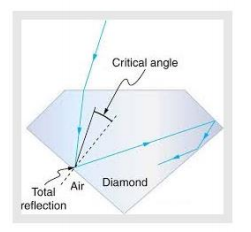The total internal reflection of the light is used in polishing diamonds to create a sparking brilliance. By polishing the diamond with specific cuts, it is adjusted the most of the light rays approaching the surface are incident with an angle of incidence more than critical angle. Hence, they suffer multiple reflections and ultimately come out of diamond from the top. This gives the diamond a sparking brilliance.

1. Light cannot easily escape a diamond without multiple internal reflections. This is because:

1. Its critical angle with reference to air is too large
2. Its critical angle with reference to air is too small
3. The diamond is transparent
4. Rays always enter at angle greater than critical angle

2. The phenomenon of Total Internal reflection occurs when

1. Light travels from rarer to denser medium
2. Light travels from denser to rarer medium
3. it does not depends on medium
4. None of these

3. The basic reason for the extraordinary sparkle of suitably cut
diamond is that

1. It has low refractive index
2. It has high transparency
3. It has high refractive index
4. It is very hard

4. A diamond is immersed in a liquid with a refractive index greater than water. Then the critical angle for total internal reflection will

1. will depend on the nature of the liquid
2. decrease
3. remains the same
4. increase

5. The following diagram shows same diamond cut in two different shapes.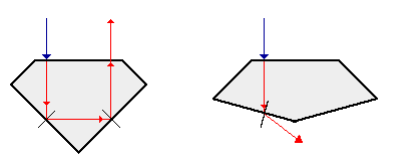The brilliance of diamond in the second diamond will be:

1. less than the first
2. greater than first
3. same as first
4. will depend on the intensity of light

A Faraday cage or Faraday shield is an enclosure made of a conducting material. The fields within a conductor cancel out with any external fields, so the electric field within the enclosure is zero. These Faraday cages act as big hollow conductors you can put things in to shield them from electrical fields. Any electrical shocks the cage receives, pass harmlessly around the outside of the cage.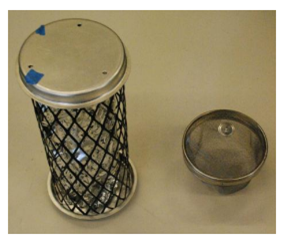1. Which of the following material can be used to make a Faraday Cage?

1. Plastic
2. Glass
3. Copper
4. Wood

2. Example of a real-world Faraday cage is

1. car
2. plastic box
3. lightning rod
4. metal rod

3. What is the electrical force inside a Faraday cage when it is struck by lightning?

1. The same as the lightning
2. Half that of the lightning
3. Zero
4. A quarter of the lightning

4. An isolated point charge +q is placed inside the Faraday cage. Its surface must have charge equal to

1. Zero
2. +q
3. –q
4. +2q

5. As the electric field inside the faraday cage is zero, what would be the electric potential inside the cage

1. zero
2. Constant
3. Increases as we move from center towards the surface of the cage
4. decreases as we move from center towards the surface of the cage

ASSERTION-REASON

For question numbers 11, 12, 13 and 14, two statements are given-one labelled Assertion (A) and the other labelled Reason (R). Select the correct answer to these questions from the codes (a), (b), (c) and (d) as given below.

1. Both A and R are true and R is the correct explanation of A
2. Both A and R are true but R is NOT the correct explanation of A
3. A is true but R is false
4. A is false and R is also false

1. Assertion: The resistivity of a semiconductor increases with temperature.

Reason: At higher temperature atoms start vibrating at higher amplitude and hence its resistivity increases.

2. Assertion: Energy of the electron hydrogen atom in first exited state is -3.4eV
Reason: Energy of electron of hydrogen atom in nth orbit is given by E=-13.6/n2

3. Assertion: Light shows the phenomenon of interference , diffraction and Polarisation.
Reason: Because of particle nature of light

4. Assertion: Conductors having equal positive charge and volume, must also have same potential.
Reason : Potential depends only on charge and volume of conductor.

5. Assertion : The induced emf and current will be same in two identical loops of copper and aluminium, when rotated with same speed in the same magnetic field.
Reason : Mutual induction does not depend on the orientation of the coils

6. Assertion : Total energy of electron in an hydrogen atom is negative.
Reason : It is bounded to the nucleus.

7. Assertion: For best contrast between maxima and minima in the interference pattern of Young’s double slit experiment, the intensity of light emerging out of the two slits should be equal.
Reason: The intensity of interference pattern is proportional to square of amplitude.

8. Assertion: If three capacitors of capacitance C1<C2<C3 are connected in parallel then their equivalent capacitance Cp>Cs
Reason :9. Assertion : An electric lamp connected in series with a variable capacitor and ac source, its brightness increases with increase in capacitance.
Reason : Capacitive reactance decrease with increase in capacitance of capacitor. in a conductor

10. Assertion: Bohr had to postulate that the electrons in stationary orbits around the nucleus do not radiate.
Reason: According to classical physical all moving electrons radiate.

11. Assertion : The drift velocity of electrons in a metallic wire will decrease, if the temperature of the wire is increased.
Reason : On increasing temperature, conductivity of metallic wire decreases.

12. Assertion: The strength of photoelectric current depends upon the intensity of incident radiation.
Reason: A photon of energy E possesses a mass equal to and momentum equal toand momentum equal to13. Assertion: A For a ray going from a denser medium to rarer medium, the ray may suffer total internal reflection.
Reason: A ray is incident from outside on a glass sphere surrounded by air as shown in figure. This ray may suffer total internal reflection at the second interface.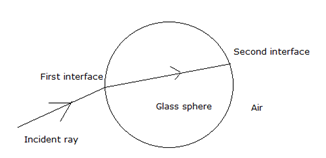14. Assertion: In a hydrogen atom, energy of emitted photon corresponding to transition from n=2 to n=1 is much greater as compared to transition from n = ∞ to  n =2
Reason: Wavelength of photon is directly proportional to the energy of emitted photon

15. Assertion:Reason: The focal length of a lens does not depend on the medium in which it is submerged.

16. Assertion : Basic difference between an electric line and magnetic line of force is that former is discontinuous and the latter is continuous or endless.
Reason :No electric lines of forces exist inside a charged body but magnetic lines do exist inside a magnet.

17. Assertion : Electric field at a point superimpose to give one resultant electric field.
Reason : Electric lines of force cross each other.

18. Assertion : If the distance between parallel plates of a capacitor is halved and dielectric constant is made three times, then the capacitor becomes 6 times. Reason : Capacity of the capacitor does not depend upon the nature of the material.

19. Asseretion: convex mirror cannot form real images.
Reason: Convex mirror converges the parallel rays that are incident on it.

20. Assertion : In Young’s double slit experiment, interference pattern disappears when one of the slits is closed.
Reason : Interference occurs due to superposition of light waves from two coherent sources

### STUDY RESOURCES

REGISTERED OFFICE : First Floor, Empire Complex, 414 Senapati Bapat Marg, Lower Parel, Mumbai - 400013, Maharashtra India.